# Test: Electrical & Electronic Measurements- 6

## 10 Questions MCQ Test RRB JE for Electrical Engineering | Test: Electrical & Electronic Measurements- 6

Description
Attempt Test: Electrical & Electronic Measurements- 6 | 10 questions in 30 minutes | Mock test for Electrical Engineering (EE) preparation | Free important questions MCQ to study RRB JE for Electrical Engineering for Electrical Engineering (EE) Exam | Download free PDF with solutions
*Answer can only contain numeric values
QUESTION: 1

### A 3 ½ digit dual slope integrated DVM, 2 V full scale is used to measure a time varying voltage V(t) = 1.0 + 2 sin 50 πt. The voltmeter indicates

Solution:

V(t) = 1 + 2 sin 50 πt
Dual slope integrated DVM measures the average value of input.
Vavg = 1 V
Hence the meter reading will be 1.000 V

*Answer can only contain numeric values
QUESTION: 2

### A digital voltmeter uses a 20 MHZ clock and has a voltage controlled generation switch provides a width of 20 μ sec per volt of unit signal. 25 V of input signal would correspond to pulse count of –

Solution: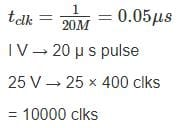*Answer can only contain numeric values
QUESTION: 3

### In a digital voltmeter, the oscillator frequency is 400 kHz and the ramp voltage falls from 8V to 0V in 20m sec. The number of pulse counted by the counter is

Solution:

Frequency = 400 kHz.
fclk = 400kHz
t = 20m sec
⇒ Clock pulses, n = t fclk = 20m sec × 400 kHz = 8000

QUESTION: 4

How would a reading 0.6973 V be displayed on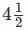digit digital voltmeter on its 10 V scale.

Solution:

Resolution on 10 V scale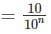N = no. of full bits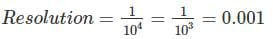Reading of 0.6973 = 0.697 volts

*Answer can only contain numeric values
QUESTION: 5

A 200 mV full scale dual-slope 3 ½ digit DMM has a reference voltage of 100 mV and a first integration time of 100 ms. For an input of [100 + 10  Cos (100πt)] mV, the conversion time (without taking the auto-zero phase time into consideration) in millisecond is______.

Solution:

Given that, Vref = 100 mV
T1 = 100 msec
in = 100 mV
We know that,
Vin T1 = Vref T2
⇒ (100) (100) = (100) (T2)
⇒ T2 = 100 msec
Total conversion time
T = T1 + T2
T = 100 + 100 = 200 ms

QUESTION: 6

A rectifier type instrument uses a bridge rectifier and has its scale calibrated in terms of rms values of a sine wave. It indicates a voltage of 2.22 V. When measuring a voltage of triangular wave shape. Estimate the error –

Solution:

The meter uses a full wave rectifier circuit it indicates value of 2.22 V the form factor for full wave rectified sinusoidal waveform is 1.11
Average value of voltage,
Vavg = 2 V
For triangular wave shape, peak value of voltage Vm = 2 Vavg = 4 V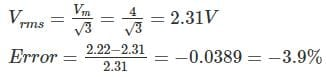*Answer can only contain numeric values
QUESTION: 7

A dual slope integrating type A/D converter has 5 μF capacitor and 25 kΩ resistor connected to it. If the reference voltage is taken as 10 V and output voltage of integrator cannot exceed 10 V then time for which reference voltage can be integrated will be – (in msec)

Solution:

The output voltage of dual slope integrating type AD converter,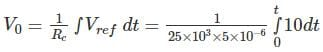= 80 t
Given that, output voltage cannot exceed 10 V
⇒ 80 t = 10 ⇒ 125 msec

QUESTION: 8

In a multimeter circuit, for a.c. voltage measurement, what is the function of diode D2?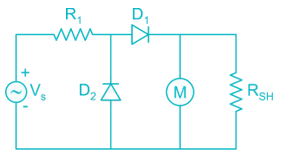Solution:

In the absence of D2, leakage current would have flowed in D1. To bypass this reverse leakage current of D1 during the negative half cycle of the input. The absence of D2 reduces the average value for complete cycle than it would have been in half wave rectification.

*Answer can only contain numeric values
QUESTION: 9

Determine the error percentage of the voltage reading due to the insertion of a voltmeter. R1 = 3 kΩ, R2 = 2 kΩ and the internal resistance of the voltmeter is 40 kΩ.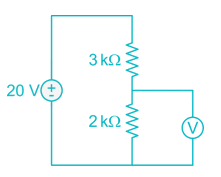Solution:

The actual voltage of R2 is: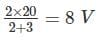The voltage after inserting the voltmeter will then be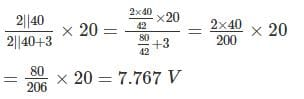Thus, error percentage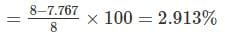QUESTION: 10

Which meter has a greater sensitivity meter A having a range of 0 - 15 V and a multiplier resistance of 22 kΩ, or meter B with a range of 0 - 300 V and multiplier resistance of 324 kΩ? Both meter moments have a resistance of 2 kΩ.

Solution:

Meter A:
Total resistance = 2 + 22 = 24 kΩ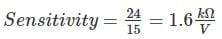Meter B:
Total resistance = 2 + 324 = 326 kΩ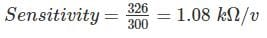Meter A is more sensitiveUse Code STAYHOME200 and get INR 200 additional OFF Use Coupon Code